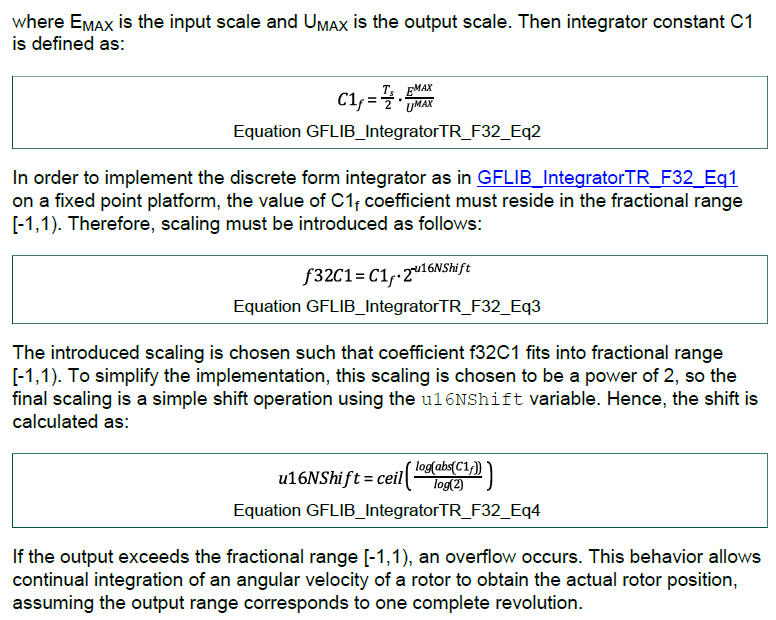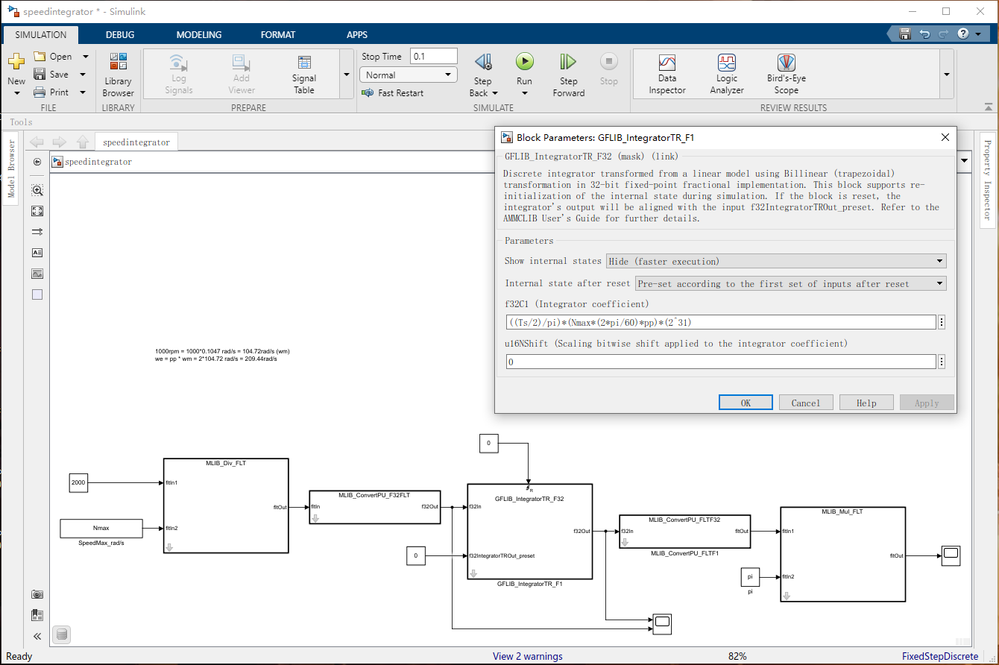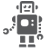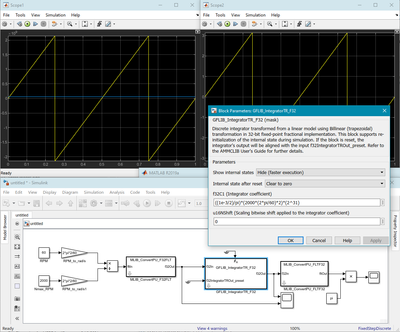## Question on GFLIB_IntegratorTR_F32 function from AMMCLIB

cancel
Showing results for
Search instead for
Did you mean:
SOLVED

## Question on GFLIB_IntegratorTR_F32 function from AMMCLIB

Jump to solution
70 ViewsContributor I

Hello, I am a little confused about the parameter setting of GFLIB_IntegratorTR_F32 function.

From the AMMCLIB manual, I get the information shown in the figure below.But the setting from the example shown here is different. Please see the picture below.Support the max motor speed is Nmax, and from the AMMCLIB manual, I know that Emax is Nmax*(2*pi/60)*pp, where pp is the pole-pairs and Emax is the max electric speed of the motor. Umax is 2^31 for the f32 format.

So I think the value for the f32C1 parameter should be (Ts/2) * (Nmax*(2*pi/60)*pp) / (2^31) * 2^(-u16NShift). And the value for u16NShift parameter should be calculated according to the equation 4.

But unfortunately, I got an error using the parameter calculation method shown above.

The setting in the second figure works, namely f32C1 is equal to ((Ts/2)/pi)*(Nmax*(2*pi/60)*pp)*(2^31) and u16NShift is equal to 0. Can anyone give a detail explanation?

Labels (1)
• ### S32 SDK for S32K1

1 Solution
35 ViewsNXP Employee

Hello,

scaling of the physical quantities ensures that the fixed-point fractional values at the input and output of the integrator are always in the fractional range [-1; 1). The C programming language does not support fractional number format so we convert our fractional numbers to their integer equivalents (by shifting the radix point after the least significant digit). Conversion between fractional and integer number format is not scaling - it is the same set of binary digits, only the radix point changed position.

The physical quantities (speed, angle) can be expressed in whatever units you want as long as you express the scaling constant in the same units.

In your example, you expressed the desired speed in mechanical revolutions per minute (RPM) and the maximum motor speed is Nmax = 2000 RPM. You then decided to recalculate the mechanical RPM to electrical radians per second (rad/s). This implies that the rotor angle will be expressed in electrical radians. Recalculation from mechanical RPM to electrical rad/s is not scaling - we still work with physical quantities, only they are now expressed in different units.

If the input to the integrator is expressed in electrical rad/s, the input scaling constant must be also expressed in el. rad/s:
Emax = Nmax*pp*2*pi/60 = 2000*2*2*pi/60 = 418.9 el. rad/s,
where pp = 2 is the number of pole pairs.

Note that 418.9 el. rad/s is the maximum speed you can feed to the integrator - in your schematic, it looks like you are feeding in 2000 el. rad/s which is beyond the maximum and therefore an invalid value.

Assume that we want to integrate the speed which is e.g. 60 RPM. This will require two steps:
1) Recalculate RPM to el. rad/s:
N_rpm = 60 RPM
N_radps = N_rpm*pp*2*pi/60 = 60*2*2*pi/60 = 12.57 el. rad/s.
2) Scale the input to the fractional range and feed it to the integrator:
f32In = N_radps/Emax = 12.57/418.9 = 0.03, which corresponds to the Q1.31 integer 64424509.

If the integrator integrates el. rad/s, the output angle will be expressed in electrical radians, i.e. the output range is [-pi, pi). To scale this range to the fractional range, we must set the output scaling constant to:
Umax = pi
the unit is the same as the output, i.e. electrical radians.

Finally, the scaled integration coefficient is:
C1f = Ts/2*Emax/Umax = Ts/2*(Nmax*pp*2*pi/60)/pi
If the coefficient value fits in the fractional range, we can set u16NShift = 0 and the above value of C1f does not need further shifting by u16NShift. The coefficient can be converted from fractional format to the Q1.31 integer format by shifting the radix point after the least significant bit:
C1f_integer = C1f*(2^31)

Attached is a screenshot of an example integrator with input speed of 60 RPM, Ts = 1 ms, the simulation runs for 1 second. We can see that the integrator output correctly completes two electrical revolutions.BR,
Petr

3 Replies
36 ViewsNXP Employee

Hello,

scaling of the physical quantities ensures that the fixed-point fractional values at the input and output of the integrator are always in the fractional range [-1; 1). The C programming language does not support fractional number format so we convert our fractional numbers to their integer equivalents (by shifting the radix point after the least significant digit). Conversion between fractional and integer number format is not scaling - it is the same set of binary digits, only the radix point changed position.

The physical quantities (speed, angle) can be expressed in whatever units you want as long as you express the scaling constant in the same units.

In your example, you expressed the desired speed in mechanical revolutions per minute (RPM) and the maximum motor speed is Nmax = 2000 RPM. You then decided to recalculate the mechanical RPM to electrical radians per second (rad/s). This implies that the rotor angle will be expressed in electrical radians. Recalculation from mechanical RPM to electrical rad/s is not scaling - we still work with physical quantities, only they are now expressed in different units.

If the input to the integrator is expressed in electrical rad/s, the input scaling constant must be also expressed in el. rad/s:
Emax = Nmax*pp*2*pi/60 = 2000*2*2*pi/60 = 418.9 el. rad/s,
where pp = 2 is the number of pole pairs.

Note that 418.9 el. rad/s is the maximum speed you can feed to the integrator - in your schematic, it looks like you are feeding in 2000 el. rad/s which is beyond the maximum and therefore an invalid value.

Assume that we want to integrate the speed which is e.g. 60 RPM. This will require two steps:
1) Recalculate RPM to el. rad/s:
N_rpm = 60 RPM
N_radps = N_rpm*pp*2*pi/60 = 60*2*2*pi/60 = 12.57 el. rad/s.
2) Scale the input to the fractional range and feed it to the integrator:
f32In = N_radps/Emax = 12.57/418.9 = 0.03, which corresponds to the Q1.31 integer 64424509.

If the integrator integrates el. rad/s, the output angle will be expressed in electrical radians, i.e. the output range is [-pi, pi). To scale this range to the fractional range, we must set the output scaling constant to:
Umax = pi
the unit is the same as the output, i.e. electrical radians.

Finally, the scaled integration coefficient is:
C1f = Ts/2*Emax/Umax = Ts/2*(Nmax*pp*2*pi/60)/pi
If the coefficient value fits in the fractional range, we can set u16NShift = 0 and the above value of C1f does not need further shifting by u16NShift. The coefficient can be converted from fractional format to the Q1.31 integer format by shifting the radix point after the least significant bit:
C1f_integer = C1f*(2^31)

Attached is a screenshot of an example integrator with input speed of 60 RPM, Ts = 1 ms, the simulation runs for 1 second. We can see that the integrator output correctly completes two electrical revolutions.BR,
Petr

15 ViewsContributor I

Hi, @petrz_

Thank you for your detailed explanation.

54 ViewsNXP Employee

Hello,

Our AMMCLIB expert is out of office.
Next week he will be available and gives you feedback.

Best regards,

Peter